﻿ Eulerian polynomials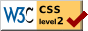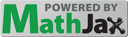# Eulerian polynomials

Peter Luschny,  2010−08−18, 2013-04-23.

The Eulerian polynomials were introduced by Leonhard Euler in his Remarques sur un beau rapport entre les séries des puissances tant directes que réciproques in 1749 (first printed in 1765) where he describes a method of computing values of the zeta function at negative integers by a precursor of Abel's theorem applied to a divergent series. The Eulerian polynomials should not be confused with the Euler polynomials.

## Definition

The Eulerian polynomials are defined by the exponential generating function

$$\sum_{n=0}^{\infty} A_{n}(t)\ \frac{x^n}{n!} = \frac{t-1}{t-\exp((t-1)x)}.$$

The Eulerian polynomials can be computed by recurrence:

$$A_{0}(t) = 1,$$ $$A_{n}(t) = t(1-t)A'_{n-1}(t)+A_{n-1}(t)(1+(n-1)t) \quad (n \ge 1)\,.$$

An equivalent way to write this definition is to set the Eulerian polynomials inductively by

$$A_{0}(t) = 1,$$ $$A_{n}(t) = \sum_{k=0}^{n-1} {n \choose k} A_{k}(t)(t-1)^{n-1-k} \quad (n \ge 1)\,.$$

The definition given is used by major authors like D. E. Knuth, D. Foata and F. Hirzebruch. In the older literature (for example in L. Comtet, Advanced Combinatorics) a slightly different definition is used, namely

$$C_{0}(t) = 1,\,$$ $$C_{n}(t) = t(1-t)C'_{n-1}(t) + C_{n-1}(t)(nt) \quad (n \ge 1)\,.$$

The sequence of Eulerian polynomials $A_n(x)$ has ordinary generating function given by the continued fraction

$$\cfrac{1}{1 - t + \cfrac{x\, t^2}{(2+x)\, t - 1 + \cfrac{4x\, t^2}{(3+2x)\, t - 1 + \cfrac{9x\, t^2}{(4+3x)\, t - 1 + \cfrac{16x\, t^2}{\cdots}}}}}$$

## Three identities

An identity due to Euler is

$$\sum_{j \ge 0} x^j(j+1)^n = \frac{A_n(x)}{(1-x)^{n+1}}.$$

For instance we get for $n\,=\,0,1,2:$

$$1 + x + x^2 + x^3 + ... = \frac{1}{1-x},$$ $$1 + 2x + 3x^2 + 4x^3 + ... = \frac{1}{(1-x)^2},$$ $$1 + 2^2 x + 3^2 x^2 + 4^2 x^3 + ... = \frac{1+x}{(1-x)^3}.$$

Let $\operatorname{S}(n,k)$ denote the Stirling numbers of the second kind. Frobenius proved that the Eulerian polynomials are equal to:

$$A_n(x) = \sum_{k=1}^{n} k! \, \operatorname{S}(n,k)(x-1)^{n-k} \quad (n \ge 1).$$

The third identity is called Worpitzky's identity

$$x^n = \sum_{0 \le k \le n } \binom{x+k}{n} [x^k] A_n(x).$$

Here $[x^k] A_n(x)$ denotes the coefficient of $x^k$ in $A_n(x)$.

## Eulerian numbers

The coefficients of the Eulerian polynomials are the Eulerian numbers $A_{n,k}$ ,

$$A_{n}(t) = \sum_{k=0}^{n} A_{n,k}\ t^{k}.$$

This definition of the Eulerian numbers agrees with the combinatorial definition in the DLMF. The triangle of Eulerian numbers is also called Euler's triangle .

 An,k 0 1 2 3 4 0 1 0 0 0 0 1 1 0 0 0 0 2 1 1 0 0 0 3 1 4 1 0 0 4 1 11 11 1 0
 Cn,k 0 1 2 3 4 0 1 0 0 0 0 1 0 1 0 0 0 2 0 1 1 0 0 3 0 1 4 1 0 4 0 1 11 11 1
 Bn,k 1 2 3 4 1 1 0 0 0 2 1 1 0 0 3 1 4 1 0 4 1 11 11 1

Euler's definition $A_{n,k}$ is A173018. The main entry for the Eulerian numbers in the OEIS database is A008292. It enumerates like $C_{n,k}$ albeit restricted to n ≥ 1 and k ≥ 1.

## The combinatorial interpretation

Let $S_n$ denote the set of all bijections (one-to-one and onto functions) from $\{1, 2, \ldots, n \}$ to itself, call an element of $S_n$ a permutation p and identify it with the ordered list $p_1p_2\ldots p_n$ .

Using the Iverson bracket [.] the number of ascents of p is defined as

$$\text{asc}(p) = \sum_{i=1}^n \left[\, p_{i} \lt p_{i+1} \, \right],$$

where pn+1 ← 0. The combinatorial interpretation of the Eulerian polynomials is then given by

$$A_{n}(x) = \sum_{p \in S_n} x^{\text{asc}(p)} .$$

The table below illustrates this representation for the case $n = 4.$

 p asc p asc p asc p asc 4321 0 4231 1 2413 2 1423 2 3214 1 2431 1 2134 2 1342 2 3241 1 4312 1 2314 2 4123 2 3421 1 3142 1 2341 2 1324 2 4213 1 4132 1 3124 2 1243 2 2143 1 1432 1 3412 2 1234 3

## HistoryLeonhard Euler introduced the polynomials in 1749  in the form

$$\sum_{k=0}^{\infty} (k+1)^{n}\ t^{k} = \frac{A_{n}(t)}{(1-t)^{n+1}}.$$

Euler introduced the Eulerian polynomials in an attempt to evaluate the Dirichlet eta function

$$\eta(s) = \sum_{n=1}^{\infty}{\frac{(-1)^{n-1}}{n^s}}$$

at $s = -1, -2, -3,\ldots$. This led him to conjecture the functional equation of the eta function (which immediately implies the functional equation of the zeta function). Most simply put, the relation Euler was after was

$$\zeta(-n) = \frac{A_{n}(-1)}{2^{n+1}-4^{n+1}} \quad (n \ge 0)\ .$$

Though Euler's reasoning was not rigorous by modern standards it was a milestone on the way to Riemann's proof of the functional equation of the zeta function. A short exposition of what Euler did was given by Keith Conrad on MathOverflow.

The facsimile shows Eulerian polynomials as given by Euler in his work Institutiones calculi differentialis, 1755. It is interesting to note that the original definition of Euler coincides with the definition in the DLMF, 2010.

## Eulerian generating functions

We call a generating function an Eulerian generating function iff it has the form

$$G_{n}(t) = \frac{g(t) A_{n}(t)}{(1-t)^{n+1}}, \quad (n \ge 0)$$

for some polynomial g(t). Many elementary classes of sequences have an Eulerian generating function. A few examples are collocated in the table below.

 n = 0 n = 1 n = 2 n = 3 n = 4 n = 5 g(t) = 1 − t2 A019590 A040000 A008574 A005897 A008511 A008512 g(t) = 1 − t A000007 A000012 A005408 A003215 A005917 A022521 g(t) = t A057427 A001477 A000290 A000578 A000583 A000584 g(t) = 1 + t A040000 A005408 A001844 A005898 A008514 A008515 g(t)=1+t+t2 A158799 A008486 A005918 A027602 A160827 A179995

For instance the case

• g(t) = t gives the generating function of the regular orthotopic numbers,
• g(t) = 1 + t gives the generating function of the centered orthotopic numbers.

## The roots of the polynomialsA1(x) ,  A2(x) ,  A3(x) ,  A4(x) ,  A5(x) ,  A6(x)

$A_n(x)$ has only (negative and simple) real roots, a result due to Frobenius. In fact the Eulerian polynomials form a Sturm sequence, that is, $A_{n+1}(x)$ has n real roots separated by the roots of $A_{n}(x)$.

## Special values

 x −1/2 1/2 3/2 2nAn(x) A179929 A000629 A004123 x −2 −1 0 An(x) A087674 A155585 A000012 x 1 2 3 An(x) A000142 A000670 A122704

## Assorted sequences and formulas

Let ∂r denote the denominator of a rational number r.

 A122778 An(n) A180085 An(−n) A000111 An(I)(1+I)(1-n) A006519 ∂(An(−1) / 2n) A001511 log2(∂(A2n+1(−1) / 22n+1))

Eulerian polynomials $A_{n}(x)$ and Euler polynomials $E_{n}(x)$ have a sequence of values in common (up to a binary shift). Let $B_{n}(x)$ denote the Bernoulli polynomials and ζ(n) the Riemann Zeta function. $\left\{{n \atop k}\right\}\,$ denotes the Stirling numbers of the second kind. The formulas below show how rich in content the Eulerian polynomials are.

 A155585 for all  $n \ge 0$ $\quad A_{n}(-1)$ $= E_{n}(1) 2^n$ $= \zeta(-n)(2^{n+1}-4^{n+1})$ $= B_{n+1}(1) \frac{4^{n+1}-2^{n+1}}{n+1}$ $= \sum_{k=0}^n \left\{ {n\atop k} \right\} (-2)^{n-k} k!$ $= \sum_{k=0}^n \sum_{v=0}^k {k \choose v} (-1)^v 2^{n-k}(v+1)^n$

## The connection with the polylogarithm

Eulerian polynomials are related to the polylogarithm

$$\text{Li}_s(z) = \sum_{k=1}^\infty {z^k \over k^s}.$$

For nonpositive integer values of s, the polylogarithm is a rational function. The first few are

 $\text{Li}_{0}(z) = {z \over 1-z};$ $\text{Li}_{-1}(z) = {z \over (1-z)^2};$ $\text{Li}_{-2}(z) = {z(1+z) \over (1-z)^3};$ $\text{Li}_{-3}(z) = {z(1+4z+z^2) \over (1-z)^4} .$

A plot of these functions in the complex plane is given in the gallery  below.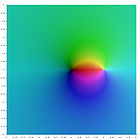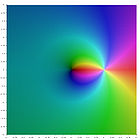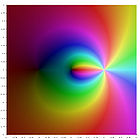$\text{Li}_{0}(z)$ $\text{Li}_{-1}(z)$ $\text{Li}_{-2}(z)$ $\text{Li}_{-3}(z)$

In general the explicit formula for nonpositive integer s is

$$\text{Li}_{-n}(z) = {{z A_n(z)} \over (1-z)^{n+1}} \qquad (n \ge 0) ~.$$

See also DLMF and the section on series representations of the polylogarithm on Wikipedia. However, note that the conventions on Wikipedia do not conform to the DLMF definition of the Eulerian polynomials.

## The connection with cardinal B-splines

The cardinal B-spline of the first order $b_1(n)$ is the characteristic function of the unit interval. The cardinal B-spline of order $n>1$ is $b_n(x) = \int_{0}^{1} b_{n-1}(x-t) \, dt$. Then for $n \gt 0$

$$A_n(x) = n! \, \sum_{k=0}^{n-1} b_{n+1}(k+1)x^k .$$

This representation of the Eulerian polynomials suggests to look also at the midpoint Eulerian polynomials

$$M_n(x) = 2^{n} n! \, \sum_{k=0}^{n} b_{n+1}(k+1/2)x^k .$$

## The midpoint Eulerian polynomials

### Generating function

The midpoint Eulerian polynomials are defined by the generating function

$$\sum_{n=0}^{\infty} \frac{M_{n}(t)}{(t-1)^n} \, \frac{x^n}{2^n n!} = \frac{t-1}{t-e^x} e^{x/2},$$

which is the counterpart to the generating function of the standard Eulerian polynomials

$$\sum_{n=0}^{\infty} \frac{A_{n}(t)}{(t-1)^n} \, \frac{x^n}{n!} = \frac{t-1}{t-e^x}.$$

### Exponential generating function

$$\sum_{n=0}^{\infty} M_{n}(x)\ \frac{t^n}{n!} = \frac{(1-x)\exp((1-x)t)}{1-x\exp(2(1-x)t)}.$$

### Recurrence relation

The midpoint Eulerian polynomials can be computed by recurrence:

$$M_{0}(t) = 1,$$ $$M_{n}(t) = 2t(1-t)M'_{n-1}(t)+M_{n-1}(t)(1+(2n-1)t) \quad (n \ge 1)\,.$$

### Expansion

The expansion analogous to Euler's given above is

$$\sum_{j \ge 0} x^j(2j+1)^n = \frac{M_n(x)}{(1-x)^{n+1}}.$$

For instance we get for $n\,=\,0,1,2:$

$$1 + x + x^2 + x^3 + ... = \frac{1}{1-x},$$ $$1 + 3x + 5x^2 + 7x^3 + ... = \frac{1+x}{(1-x)^2},$$ $$1 + 3^2 x + 5^2 x^2 + 7^2 x^3 + ... = \frac{1+6x+x^2}{(1-x)^3}.$$

### Worpitzky-type identity

$$(2x+1)^{n} = \sum_{0 \le k \le n } \binom{x+k}{n} [x^k] M_n(x).$$

### Roots of the polynomials

$M_n(x)$ has n zeros which are simple and negative.

### The midpoint Eulerian numbers

The coefficients of the midpoint Eulerian polynomials are the midpoint Eulerian numbers $M_{n,k}$

$$M_{n}(t) = \sum_{k=0}^{n} M_{n,k}\ t^{k}.$$
 Mn,k 0 1 2 3 4 row sum 0 1 0 0 0 0 1 1 1 1 0 0 0 2 2 1 6 1 0 0 8 3 1 23 23 1 0 48 4 1 76 230 76 1 384

### The combinatorial interpretation

Let $B_n$ denote the set of signed permutations of $$I = \{ \pm i \ : \ 1 \le i \le n \}$$ such that $p(-i) = -p(i)$ for all $i \in I$. The descent number of $p$ is defined as $$des(p) = \text{card} \{i \in [n]: p(i-1) \gt p(i)\}$$ where $p(0) = 0$. Then $$M_n(x) = \sum_{p \in B_n} x^{des(p)}.$$

The table below illustrates this representation for the case $n = 2.$

 p des p des -2, -1, 1, 2 0 1, -2, 2, -1 1 -2, 1, -1, 2 1 1, 2, -2, -1 1 -1, -2, 2, 1 1 2, -1, 1, -2 1 -1, 2, -2, 1 1 2, 1, -1, -2 2

## Program

(Maple)
a := proc(n, m) local k; # Eulerian numbers
end:
A := proc(n, x) local k; # Eulerian polynomials
end:
ma := proc (n, m) local k; # Midpoint Eulerian numbers
end:
mr := proc(n, k) option remember; # Recursive mid. Eul.num.
if n = 0 then if k=0 then 1 else 0 fi else
(2*(n-k)+1)*mr(n-1, k-1) + (2*k+1)*mr(n-1, k) fi
end:
MA := proc(n, x) local k; # Midpoint Eulerian polynomials
end:
B := proc(n, u) # Cardinal B-splines
if n = 1 then if (u < 0) or (u >= 1) then 0 else 1 fi
else (u/(n-1))*B(n-1, u)+((n-u)/(n-1))*B(n-1, u-1) fi
end:

(Sage)
def a(n, m) :  # Eulerian numbers
return add((-1)^k*binomial(n+1, k)*(m+1-k)^n for k in (0..m))

def A(n, x) :  # Eulerian polynomials
return add(a(n, k)*x^k for k in (0..n))

def ma(n, m):  # Midpoint Eulerian numbers
return add((-1)^(m-k)*binomial(n+1, m-k)*(2*k+1)^n for k in (0..m))

#CachedFunction
def mr(n, k) : # Recursive midpoint Eulerian numbers
if n == 0: return 1 if k == 0 else 0
return (2*(n-k)+1)*mr(n-1, k-1) + (2*k+1)*mr(n-1, k)

def MA(n, x):  # Midpoint Eulerian polynomials
return add(mr(n, k)*x^k for k in (0..n))

def B(n, x):   # Cardinal B-splines
if n == 1: return 0 if (x < 0) or (x >= 1) else 1
return (x/(n-1))*B(n-1, x)+((n-x)/(n-1))*B(n-1, x-1)


## Notes

1. The Eulerian number $A_{n,k}$ is not to be confused with the value of the nth Eulerian polynomial at k. For instance $A_{n,n} = 1,0,0,0,\ldots$ whereas $A_{n}(n)$ is A122778.
2. Digital Library of Mathematical Functions, National Institute of Standards and Technology, Table 26.14.1
3. The name Euler's triangle is used, for example, in Concrete Mathematics, Table 254. A virtue of this name is that it might evoke an association to Pascal's triangle, with which it shares the symmetry between left and right.
4. Euler read his paper in the Königlichen Akademie der Wissenschaften zu Berlin in the year 1749 ("Lu en 1749"). It was published only much later in 1768.
5. Author of the plots of the polylogarithm functions in the complex plane: Jan Homann. Public domain.

## References

Parts of this article were originally written for the OeisWiki. Thanks to Daniel Forgues for editorial help.